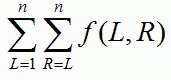Competitions

# Intervals on Tree

We have a tree with n vertices and n1 edges, respectively numbered 1, 2, ..., n and 1, 2, ..., n1. Edge i connects vertices ui and vi.

For integers L, R (1LRn), let us define a function f(L, R) as follows:

• Let S be the set of the vertices numbered L through R. f(L, R) represents the number of connected components in the subgraph formed only from the vertex set S and the edges whose endpoints both belong to S.

Compute#### Input

The first line contains number n (1n2 * 105). Each of the next n - 1 lines contains two vertices (ui, vi) (1ui, vin) - an edge in the graph.

#### Output

Print the value of the sum.

#### Example

We have six possible pairs (L, R) as follows:

For L = 1, R = 1, S = {1} and we have 1 connected component.

For L = 1, R = 2, S = {1, 2} and we have 2 connected components.

For L = 1, R = 3, S = {1, 2, 3} and we have 1 connected component, since S contains both endpoints of each of the edges 1, 2.

For L = 2, R = 2, S = {2} and we have 1 connected component.

For L = 2, R = 3, S = {2, 3} and we have 1 connected component, since S contains both endpoints of Edge 2.

For L = 3, R = 3, S = {3} and we have 1 connected component.

The sum of these is 7.

Time limit 1 second
Memory limit 128 MiB
Input example #1
3
1 3
2 3

Output example #1
7